# While loop with Compile time constants

• Last Updated : 14 Dec, 2020

While loop is a control flow statement that allows code to be executed repeatedly based on a given Boolean condition. The while loop can be thought of as a repeating if statement. It is mostly used in situations where the exact number of iterations beforehand. Below is the image to illustrate the while loop: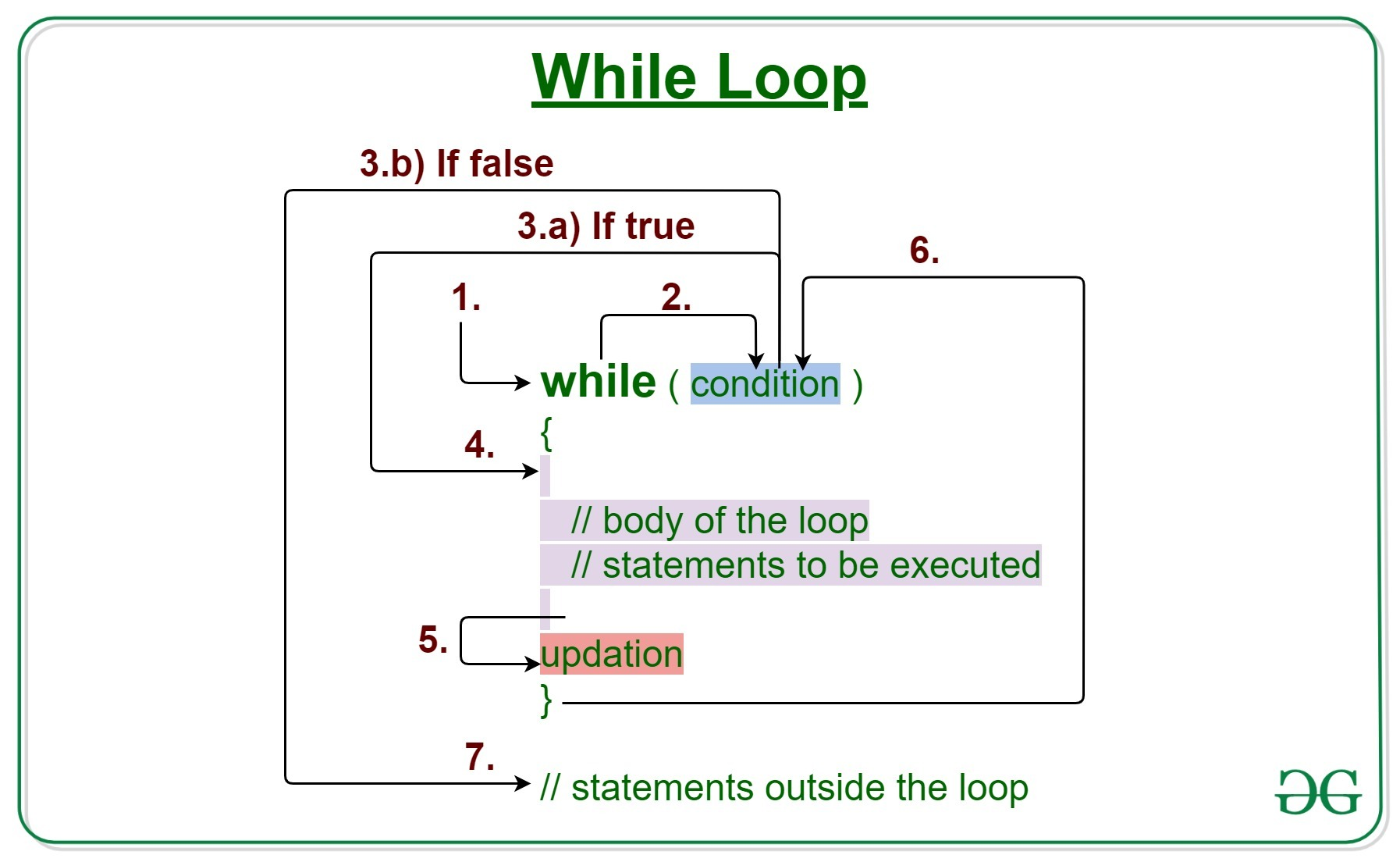Syntax:

```while(test_expression)
{
// statements
update_expression;
}```

Program 1: Below is the program to implement the basic while loop:

## Java

 `// Java program to illustrate the``// while loop`` ` `class` `GFG {`` ` `    ``// Driver Code``    ``public` `static` `void` `main(String args[])``    ``{``        ``// Initialization expression``        ``int` `i = ``1``;`` ` `        ``// Test expression``        ``while` `(i < ``6``) {``            ``System.out.println(``"Hello World"``);`` ` `            ``// Update the expression``            ``i++;``        ``}``    ``}``}`

Output:

```Hello World
Hello World
Hello World
Hello World
Hello World
```

### While Loop With Boolean Value

Program 1: Below is the example of another while Loop where a boolean variable is used in the test_expression part:

## Java

 `// Java program to demonstrate while loop``import` `java.io.*;`` ` `class` `GFG {``    ``// Driver Code``    ``public` `static` `void` `main(String[] args)``    ``{``        ``boolean` `flag = ``false``;`` ` `        ``while` `(flag) {``            ``System.out.println(``                ``"While Loop Executed"``);``        ``}`` ` `        ``System.out.println(``"Done!"``);``    ``}``}`

Output:

```Done!
```

Explanation: The above code works fine because the flag is not a compile-time constant.  The value of the flag is false so the while loop is not be executed.

Program 2: Below is the example of a while loop where a constant boolean value is used in the test_expression part instead of using a boolean variable as done in the above example.

## Java

 `// Java program to demonstrate the``// above statement`` ` `import` `java.io.*;``class` `GFG {``    ``// Driver Code``    ``public` `static` `void` `main(String[] args)``    ``{``        ``while` `(``false``) {``            ``System.out.println(``                ``"While Loop Executed"``);``        ``}``        ``System.out.println(``"Done!"``);``    ``}``}`

Output: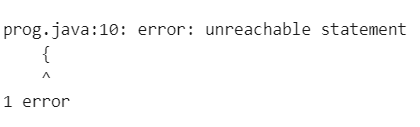Explanation: The above code fails to compile as the code inside while loop is unreachable.

Program 3: Below is the program to demonstrate the scenario when the test_expression is “true”:

## Java

 `// Java program to demonstrate the``// above statement``import` `java.io.*;`` ` `class` `GFG {`` ` `    ``// Driver Code``    ``public` `static` `void` `main(String[] args)``    ``{``        ``while` `(``true``) {``            ``System.out.println(``                ``"While Loop Executed"``);``        ``}``        ``System.out.println(``"Done!"``);``    ``}``}`

Output: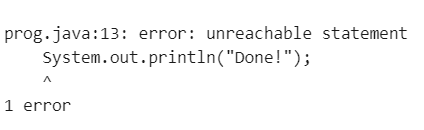Explanation: In the above code, the while loop will be compiled successfully, but it would end up in an infinite loop and because of that the code after the while loop is unreachable. Therefore, it would also end up compilation error.

Program 4: Below is another version of the above program with a break statement inside the while loop:

## Java

 `// Java program to demonstrate the``// above approach``import` `java.io.*;`` ` `class` `GFG {`` ` `    ``// Driver Code``    ``public` `static` `void` `main(String[] args)``    ``{``        ``while` `(``true``) {``            ``System.out.println(``                ``"Inside Loop"``);``            ``break``;``        ``}``        ``System.out.println(``"Done!"``);``    ``}``}`

Output:

```Inside Loop
Done!
```

Explanation: In the above program, if the while loop contained a break statement then the compilation will not fail because there would be some cases where the code after the loop would be reachable. Here, we are coming out of the while loop and the print statement will be executed.

### Compile-time Constants:

Compile-time constants are the constants whose respective values are known at compile time. Final variables of primitive data types and strings can be compile-time constants. They must be initialized with their declaration. Below is an example of compile-time constants:

```final int i = 100;
final String str = "Hello";```

Following are not compile-time constants:

```final int j;
j = 10;
final Integer k = 20;```

Program 1: Suppose a final variable as a condition variable inside the while loop is used. Below is the program to illustrate the same:

## Java

 `// Java program to demonstrate the``// while loop with compile-time``// constant``import` `java.io.*;`` ` `class` `GFG {`` ` `    ``// Driver Code``    ``public` `static` `void` `main(String[] args)``    ``{``        ``final` `boolean` `flag = ``false``;`` ` `        ``while` `(flag) {``            ``System.out.println(``                ``"Inside Loop"``);``        ``}``        ``System.out.println(``"Done!"``);``    ``}``}`

Output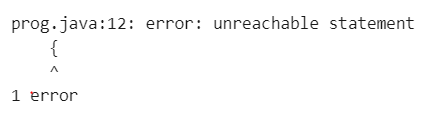Explanation: As the flag is a final variable, it is a compile-time constant. As the value of the flag cannot be changed, the code inside the while loop is unreachable. That is why this fails to compile.

Program 2: If the final variable is not initialized when it is declared, then it is not a compile-time constant. Below is the program to illustrate the same:

## Java

 `// Java program to demonstrate the``// above statement``import` `java.io.*;`` ` `class` `GFG {`` ` `    ``// Driver Code``    ``public` `static` `void` `main(String[] args)``    ``{``        ``final` `boolean` `flag;``        ``flag = ``false``;`` ` `        ``while` `(flag) {``            ``System.out.println(``"Inside Loop"``);``        ``}``        ``System.out.println(``"Done!"``);``    ``}``}`

Output:

```Done!
```

Explanation: In the above program, there would not be any compilation error as the flag is not initialized when it is declared. It is initialized on a separate line. So in this case flag is not a compile-time constant and there would not be any compilation error.

Note: Similarly, if the value of the final flag variable is true and it is initialized when it is declared, then the code after the while block will fail to compile. But, if the value of the final boolean flag is initialized on a separate line with the value ‘true’, then there would not be any compilation error. That means, if the condition variable used inside the while loop is a compile-time constant, then the code may or may not compile depending on the context. The final variable that is not initialized on the same line is not a compile-time constant. So in that case there is no issue.

Program 3:

## Java

 `// Java program to demonstrate the while``// loop with compiled time constant``import` `java.io.*;`` ` `class` `GFG {`` ` `    ``// Driver Code``    ``public` `static` `void` `main(String[] args)``    ``{``        ``boolean` `anotherFlag = ``false``;``        ``final` `boolean` `flag = anotherFlag;`` ` `        ``// While loop``        ``while` `(flag) {``            ``System.out.println(``"Inside Loop"``);``        ``}``        ``System.out.println(``"Done!"``);``    ``}``}`

Output:

```Done!
```

Explanation: In the above code, there would not be any compilation error, though the flag is a final variable and it is initialized on the same line, ‘anotherFlag’ is not a final variable and that’s why the flag is not a compile-time constant and thus there are no issues with the compilation.

Program 4: If the ‘anotherFlag’ was a final variable, then the results would have been different. Below is the program to illustrate the same:

## Java

 `// Java program to demonstrate the``// above statement``import` `java.io.*;`` ` `class` `GFG {`` ` `    ``// Driver Code``    ``public` `static` `void` `main(String[] args)``    ``{``        ``final` `boolean` `anotherFlag = ``false``;``        ``final` `boolean` `flag = anotherFlag;`` ` `        ``while` `(flag) {``            ``System.out.println(``"Inside Loop"``);``        ``}``        ``System.out.println(``"Done!"``);``    ``}``}`

Output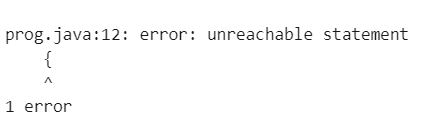Explanation: In the above code, the flag is a compile-time constant. So, the code inside the while loop is unreachable, resulting in the compilation error.

My Personal Notes arrow_drop_up